Geometry 8-2 Special Right Triangles
starstarstarstarstarstarstarstarstarstar
by Matthew Richardson
| 26 Questions
Note from the author:
A complete formative lesson with embedded slideshow, mini lecture screencasts, checks for understanding, practice items, mixed review, and reflection. I create these assignments to supplement each lesson of Pearson's Common Core Edition Algebra 1, Algebra 2, and Geometry courses. See also mathquest.net and twitter.com/mathquestEDU.
The outlined content above was added from outside of Formative.
The outlined content above was added from outside of Formative.1
1
10 pts
Solve It! This map of part of a college campus shows a square "quad" area with walking paths. The distance from the dorm to the dining hall is 150 yd. Suppose you go from your dorm to the dining hall, to the science lab, to your dorm, to the student center, to the library, and finally back to your dorm. To the nearest tenth, how far do you walk? (Assume you always take the most direct route and stay on the paths.)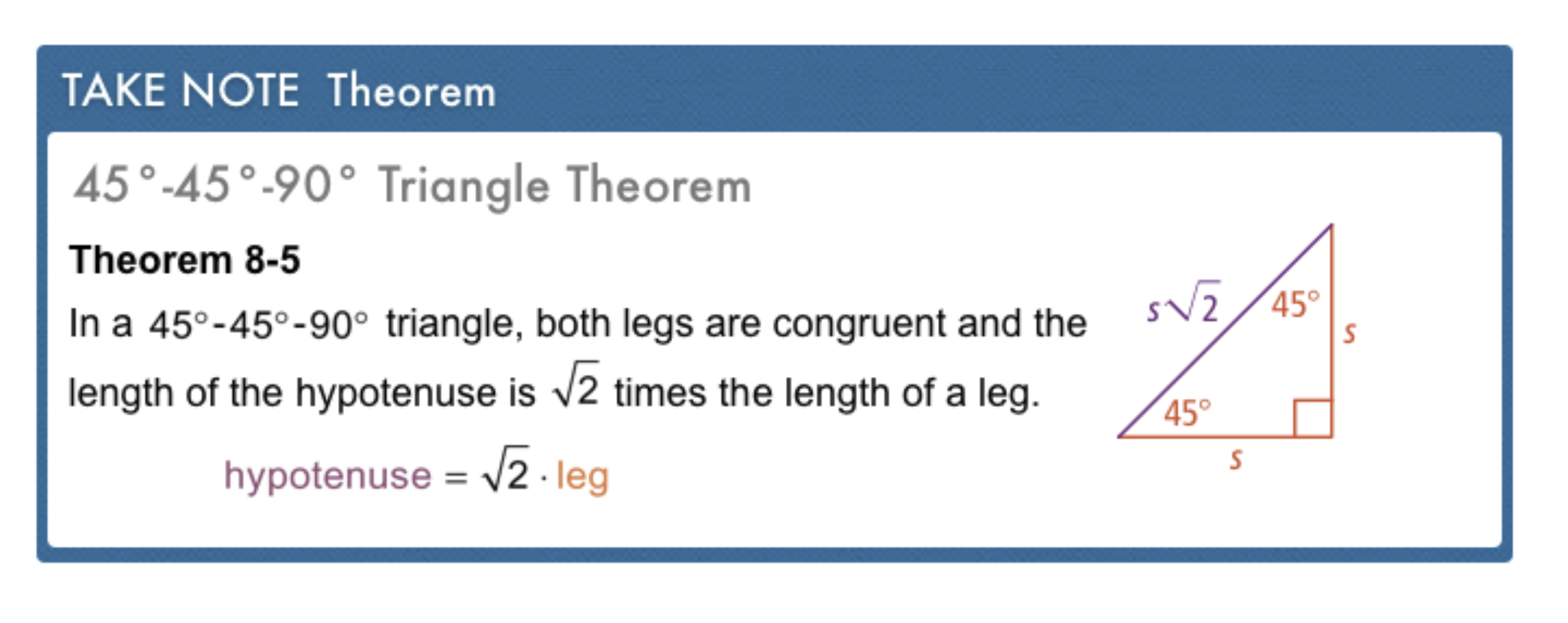The outlined content above was added from outside of Formative.2
2
10 pts
Problem 1 Got It?
A
B
C
D
The outlined content above was added from outside of Formative.3
3
10 pts
Problem 2 Got It?
A
B
C
D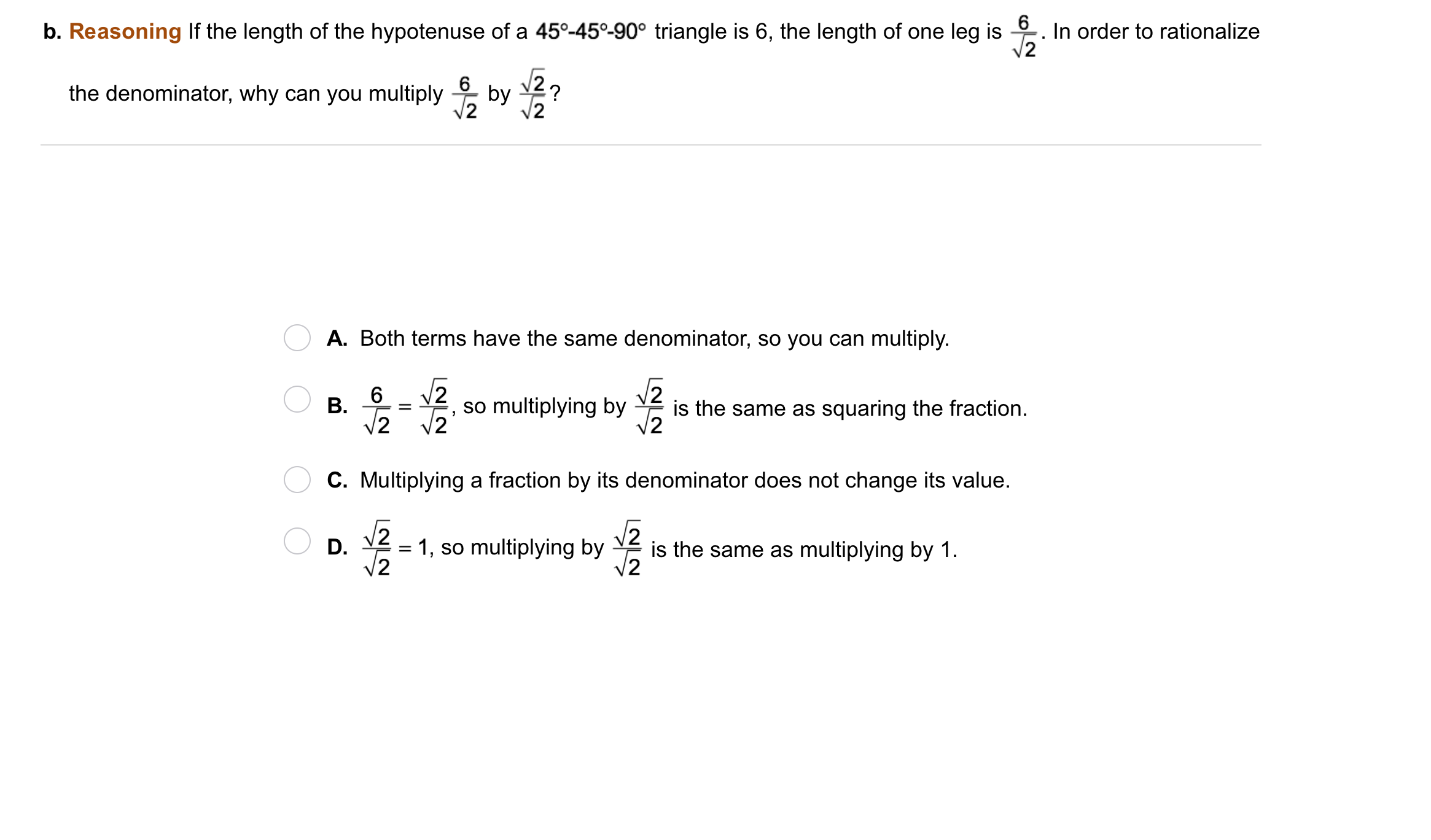4
4
10 pts
Problem 2 Got It?
A
B
C
D
The outlined content above was added from outside of Formative.5
5
10 pts
Problem 3 Got It?
A
B
C
D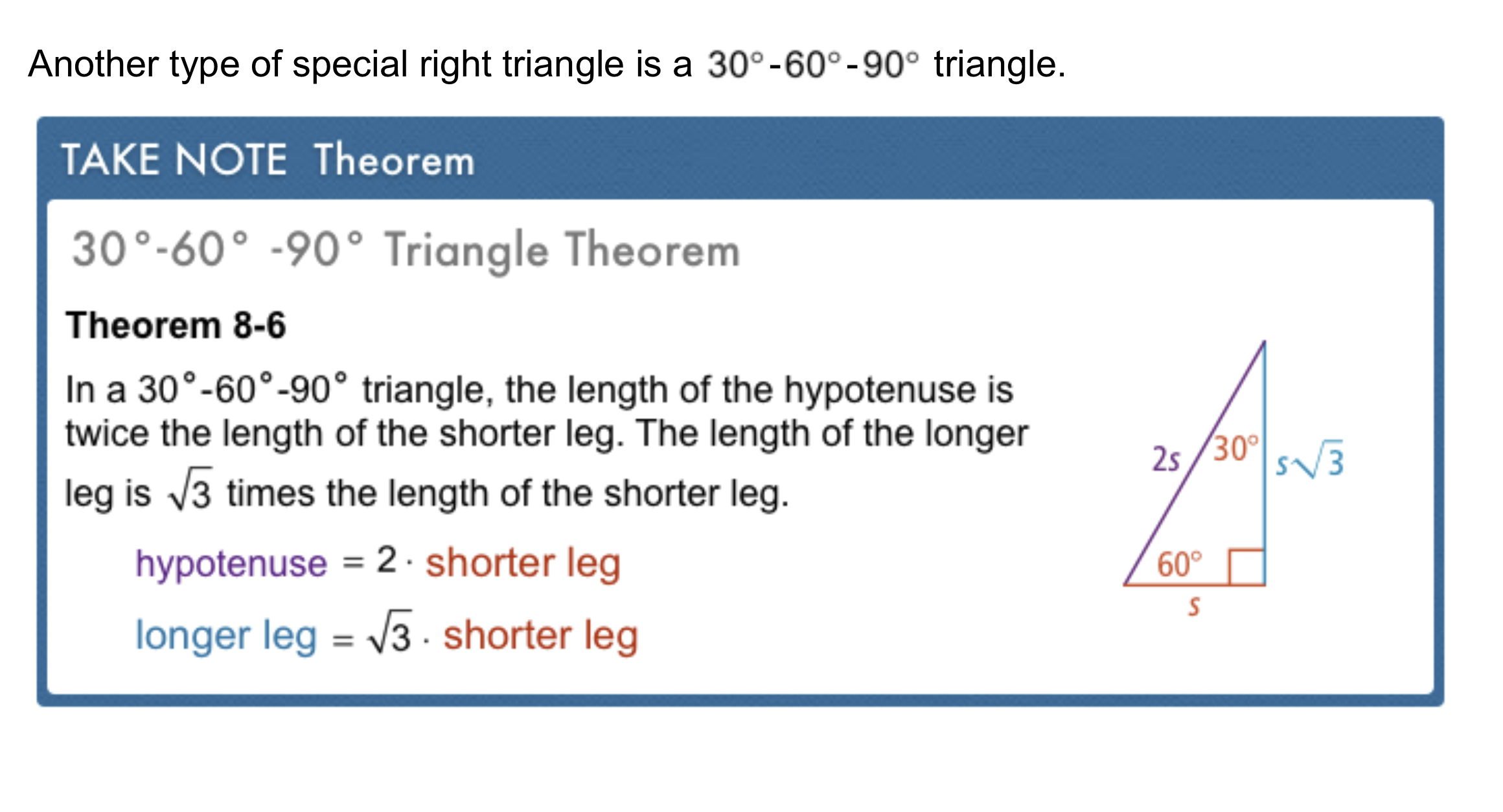The outlined content above was added from outside of Formative.6
6
10 pts
Problem 4 Got It?
A
B
C
D
The outlined content above was added from outside of Formative.7
7
10 pts
Problem 5 Got It?
A
B
C
D8
8
10 pts
A
B
C
D9
9
10 pts
A
B
C
D10
10
10 pts
A
B
C
D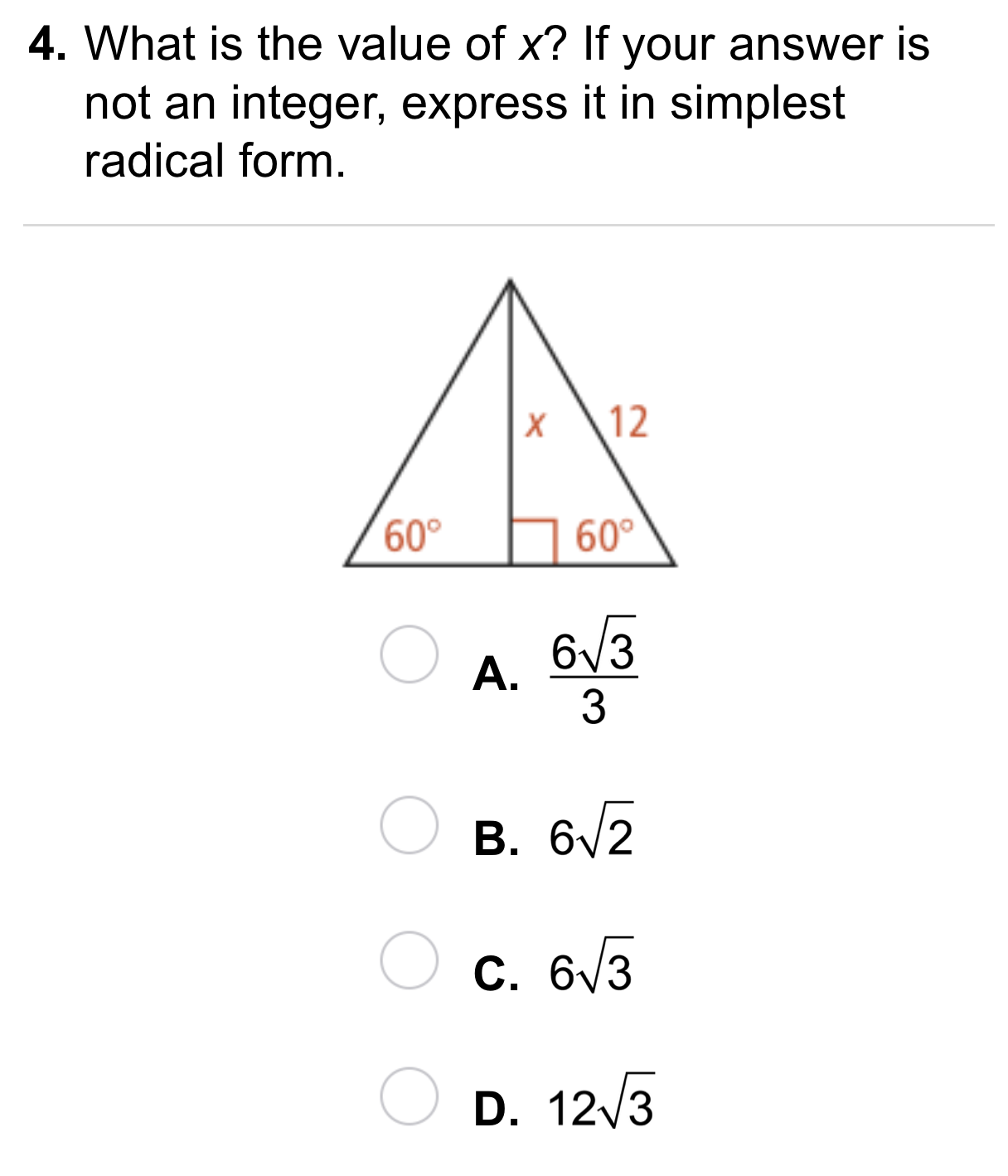11
11
10 pts
A
B
C
D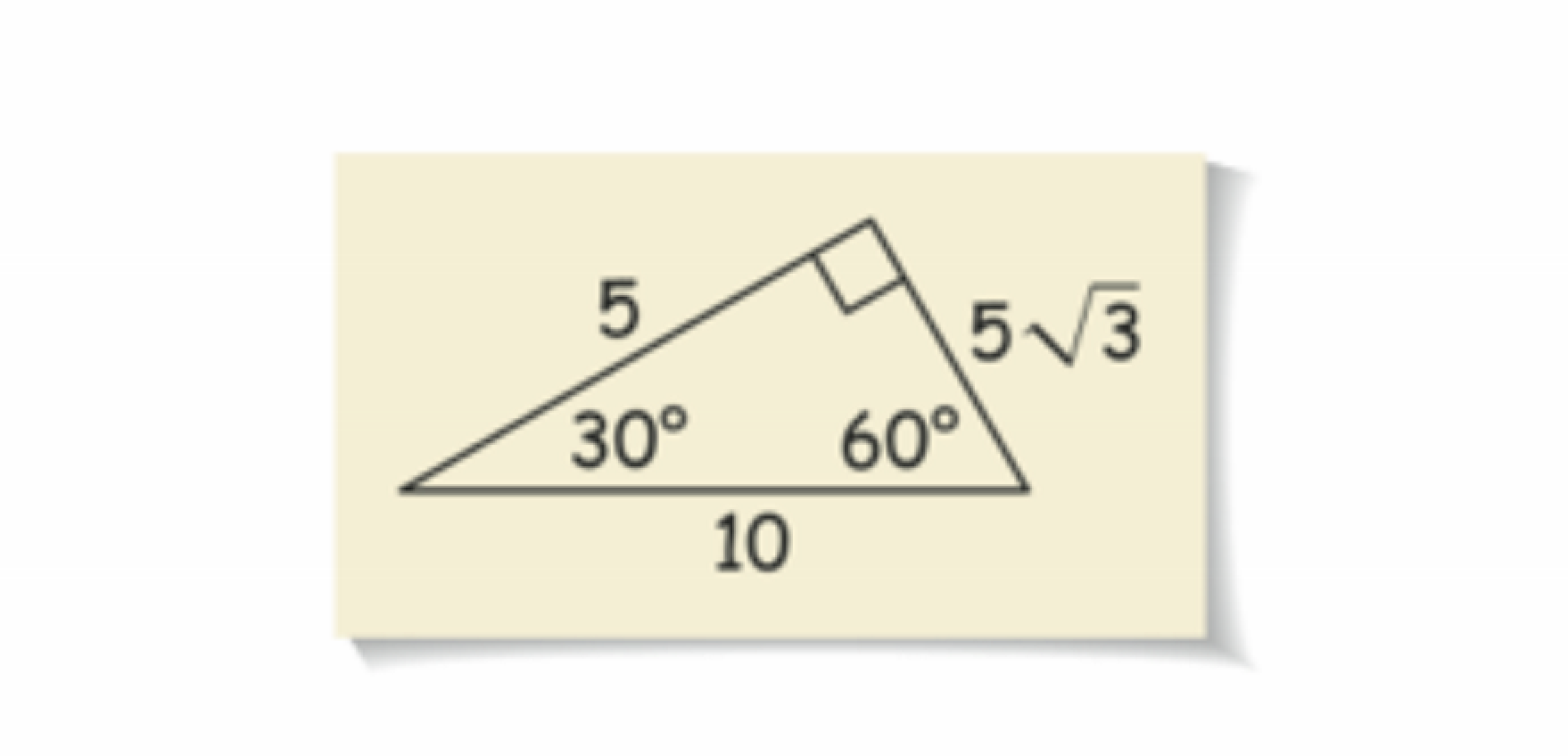12
12
10 pts
Error Analysis: Sandra drew the triangle below. Rika said that the labeled lengths are not possible. With which student do you agree? Explain.
13
10 pts
Reasoning: A test question asks you to find two side lengths of a 45-45-90 triangle. You know that the length of one leg is 6, but you forgot the special formula for 45-45-90 triangles. Explain how you can still determind the other side lengths. What are the other side lengths?14
10 pts
Review Lesson 8-1: A right triangle has a 6-in. hypotenuse and a 5-in. leg. Find the length of the other leg in simplest radical form.

15
10 pts
Review Lesson 7-1: Solve each proportion.

Match the solutions on the left with the proportions on the right. Solutions are written as reduced fractions or decimals rounded to the nearest hundredth. Not all solutions will be used. Not all proportions will be solved.
• x = 1.71
• x = 60/7
• x = 4.90
• x = 15/2
• x = 11/53
• Proportion A
• Proportion B
• Proportion C
• Proportion D16
16
10 pts
Vocabulary Review: Which segment is a diagonal of square ABCD?
segment AB
segment AC
segment BC
segment CD17
5 pts
Use Your Vocabulary: Complete the statement with the word complement or complementary. Write only the word.

If m∠A = 40 and m∠B = 50, the angles are __?__.
18
5 pts
Use Your Vocabulary: Complete the statement with the word complement or complementary. Write only the word.

If m∠A = 30 and m∠B = 60, ∠B is the __?__ of ∠A.
19
5 pts
Use Your Vocabulary: Complete the statement with the word complement or complementary. Write only the word.

∠P and ∠Q are __?__ because the sum of their measures is 90.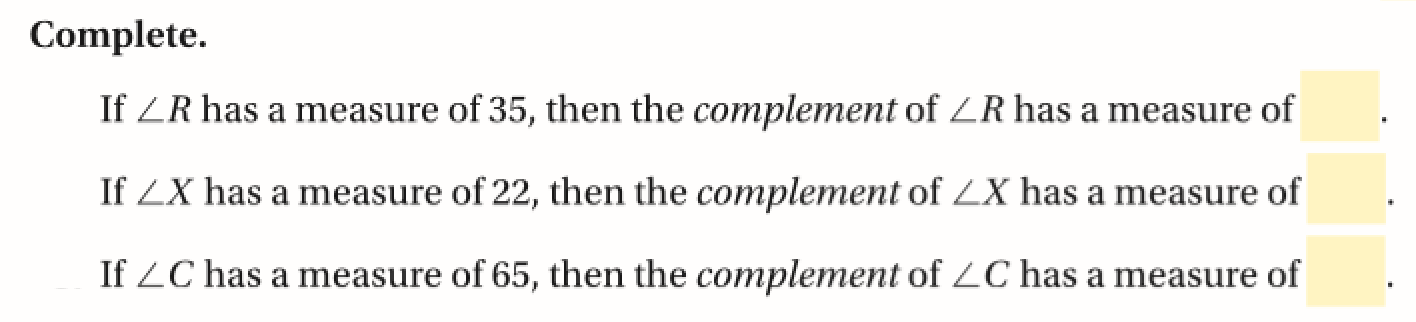20
21
22
20
5 pts
Enter only a number.
21
5 pts
Enter only a number.
22
5 pts
Enter only a number.
23
10 pts
Use Your Vovabulary: Select a pair of complementary angles from the angle measurements below.
60
40
50
120
24
10 pts
Theorem 8-5: Fill in the three blanks on the canvas to demonstrate your understanding of the 45-45-90 triangle theorem.
25
10 pts
Theorem 8-6: Fill in the two blanks on the canvas to demonstrate your understanding of the 30-60-90 triangle theorem.
26
10 pts
Reflection: Math Success